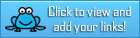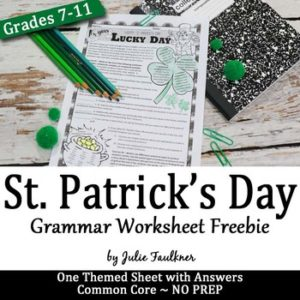# Daily Archives: February 12, 2018

## February Teacher Talk

Posted by Deann Marin of Socrates Lantern

Welcome to our February Teacher Talk.  All of us from the Teacher Talk collaborative would like wish you a Happy Valentines Day.  We have so many fab things this month from  proof-reading ideas, to ELA to math activities, to celebrating Black History Month and President’s Day, you don’t want to miss reading these blog posts from some awesome educators. This month, we’re honored to have Daniel with us, as our  guest blogger.
If you’re interested in joining this unique group of teacher entrepreneurs and blogging buddies and our blog linky, sign up here…. If you decide to join, be sure to mention one of our names.
<<<<<<<<<<<<<<<<<<<<<<<<<<<<<<<<<<<<<<<<<<<<<<<<<<<<<<<<<<<
Navigating Life as a Single Dad
By Daniel of Dad Solo whose guest post can be found at Rainbow City Learning

Single parents have a difficult job, and even in the best of circumstances, it can be a struggle. Moms have it tough, but so do dads.
<<<<<<<<<<<<<<<<<<<<<<<<<<<<<<<<<<<<<<<<<<<<<<<<<<<<<<<<<<<
I Dreaded my Third Period Class
By Deann Marin of Socrates Lantern

It was cold and blustery outside, one of those days that you just wanted to stay under the covers. But it was only hump day and I had a busy schedule, so I dragged myself out of bed, had my cup of coffee and a piece of toast, jumped into my car and was on my way.
<<<<<<<<<<<<<<<<<<<<<<<<<<<<<<<<<<<<<<<<<<<<<<<<<<<<<<<<<<<

Is This Routine? Why Not?
By Susan Berkowitz of Susan Berkowitz

Research tells us that routines are the heart of language and symbbol development. Routines are a sequence of actions or events that are repeated over and over again.
<<<<<<<<<<<<<<<<<<<<<<<<<<<<<<<<<<<<<<<<<<<<<<<<<<<<<<<<<<<

Add Projects to Your Bag of Tricks!
By Sally Hansen of Purposeful Plans

Have you ever set a goal to cover everything you are required to teach during the school year?  Many teachers try to meet this goal each year and end up frustrated because we are short on time!  It is very difficult to get everything we are required to teach during the school year, take the cross-curricular approach!
<<<<<<<<<<<<<<<<<<<<<<<<<<<<<<<<<<<<<<<<<<<<<<<<<<<<<<<<<<<

Improving Class Cooperation
By Gini Musmanno of Reading Spotlight

Wishing and hoping, and thinking and praying, for a courteous and amenable class? Then give students a little nudge toward cooperation and affirmation of each other this month.
<<<<<<<<<<<<<<<<<<<<<<<<<<<<<<<<<<<<<<<<<<<<<<<<<<<<<<<<<<<

Amazon Alexa in Your Classroom
By Lisa Robles of LisaTeachR’s Classroom

Learn about using Amazon Alexa in your classroom.
<<<<<<<<<<<<<<<<<<<<<<<<<<<<<<<<<<<<<<<<<<<<<<<<<<<<<<<<<<<

Building Understanding, One Brick at a Time
By Kathie Yonemura of Tried & True Teaching Tools

Getting your hands muddy is a great way to learn! Social Studies is meaningful when students experience content learned.
<<<<<<<<<<<<<<<<<<<<<<<<<<<<<<<<<<<<<<<<<<<<<<<<<<<<<<<<<<<

How to Select Books for Your Classroom Book Clubs
By Marcy Howe of It’s a Teacher Thing

Read five essential tips for finding “just right” books for your classroom book clubs. The books you choose are crucial to running successful classroom book clubs.
<<<<<<<<<<<<<<<<<<<<<<<<<<<<<<<<<<<<<<<<<<<<<<<<<<<<<<<<<<<
The Fine Motor Advantage
By Thia Triggs of Print Path

What does research tell us about the impact of fine motor skills on early academics?
<<<<<<<<<<<<<<<<<<<<<<<<<<<<<<<<<<<<<<<<<<<<<<<<<<<<<<<<<<<## Free: Poetry Through Song – Individual Lesson (Sound of Silence)

https://www.teacherspayteachers.com/Product/Poetry-Through-Song-Individual-Lesson-Sound-of-Silence-3638399Product Description
This individual 2-day lesson teaches poetry through song lyrics

The lesson included in this mini bundle includes a lesson plan, student activity sheets, and a comprehensive answer key. Students will analyze the song “The Sound of Silence,” will learn the importance of allusions, will learn about theme development, and will compare both the original Simon and Garfunkle version to the Disturbed version, all while furthering the theme of the song.

Poetry should be fun, and it can be!

This lesson is a preview of my unit called “Poetry Through Song.” Check out the preview to the unit here:
Poetry Through Song Unit
You may also be interested in the following products:
Lord of the Flies Unit (No prep required)
Hamlet Unit (No prep – Complete Unit)
Death of a Salesman (No prep required)
Frankenstein (No prep – Complete Unit)
Poetry Unit for Senior Students (NO PREP)
Poetry Unit for Intermediate Students
Poetry Unit: The Art of Interpretation
Romeo and Juliet – A No Prep Unit
It’s teaching made easy!

IF YOU ARE NOT ABLE TO OPEN THE PDF FILE, PLEASE

E-MAIL ME at teachwithpassion101@gmail.com . Be sure to include your e-mail address and buyer’s name. I will send you an attachment directly to your e-mail address.

Total Pages
7 pages
Answer Key
Included
Teaching Duration
2 days

## FREE LANGUAGE ARTS LESSON – “St. Patrick’s Day Grammar Worksheet, NO PREP, Middle and High School, FREEBIE”

by Julie Faulkner

8th – 12th GradeIt’s Your Lucky Day – A St. Patrick’s Day Freebie!!

This grammar worksheet is a sampling of my seasonal grammar worksheets that aren’t your typical grammar exercises with sentences about the holiday! They are grammar challenges that reflect the seasonally-inspired thematic concept of the page. I’ve also provided beautiful clip art to add layers and interest to the pages to grab your teens’ eyes! Plus, for those fast finishers, there’s plenty for them to color and style! Extension activities are also provided on several of the sheets, and the grammar notes are included as well. Each worksheet covers a different – and single – grammar skill.

Skill/Theme Included on this SAMPLE WORKSHEET:
It’s Your Lucky Day: Four Types of Sentences Structures

Suggested Uses:
Traditional grammar practice
Sub sheets
Literacy centers
Learning stations
Homework
Test Prep
Review

WAIT! See my seasonal grammar worksheets full-year BUNDLE!
Seasonal Grammar Worksheets BUNDLE

Other teachers who’ve tried my seasonal grammar worksheets shared these classroom success stories:
♥ “This was a fun activity for my SAT Prep class. The examples were great for high school – they weren’t too elementary for 10th and 11th graders. They enjoyed the examples and I liked that they weren’t too easy and weren’t too hard – great for review and discussion.”
♥ “These were so fun to use, and with so many options, I was able to use some with one level and some with another which helped since I have multiple kids taking both classes and I hate to have them do the same thing!”
♥ “Another excellent product from Team Faulkner. Great product. A true time saver.”

Be the first to know about my new discounts, freebies and product launches. Look for the green star next to my store logo and click it to FOLLOW ME. Presto! You will now receive email updates about my store.

Original Cover Photography © Julie Faulkner Photography

https://www.teacherspayteachers.com/Product/St-Patricks-Day-Grammar-Worksheet-NO-PREP-Middle-and-High-School-FREEBIE-3052510

Join The Best of Teacher Entrepreneurs Marketing Cooperative at
http://www.thebestofteacherentrepreneursmarketingcooperative.com/2014/01/the-best-of-teacher-entrepreneurs.html
and get THOUSANDS OF PAGE VIEWS for your TpT products!

Go to http://www.pinterest.com/TheBestofTPT/ for even more free products!